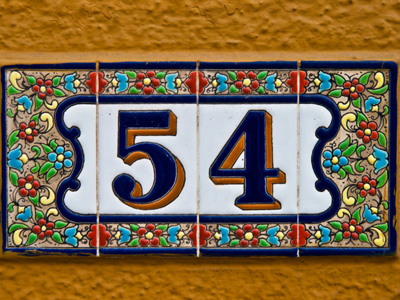34 + 15 + 6 - 1 = 54.

# Addition and Subtraction (Very Easy)

## Quiz About Plus and Minus

Addition and subtraction sums include the plus or minus sign. If you are worried about your addition and subtraction - don't be! With practice, they are very easy operations to carry out.

In this first quiz, you'll get some practice with dealing with really easy numbers. TIP: With numbers less than 100, add the tens first and then add the ones. For example:

• 12 + 16 + 22 = (10 + 10 + 20) + (2 + 6 + 2) = 40 + 10 = 50

Play this 11-plus Maths quiz and if you get full marks, move onto the next Very Easy quiz in this series, and so on. Or you could move up to our Easy quizzes, for more of a challenge.When you've got all the answers correct ~ without using a pencil and paper ~ then you will have a really good grasp of addition and subtraction.

1.
12 + 12 + 12 = ?
35
34
36
32
12 + 12 + 12 = (10 + 10 + 10) + (2 + 2 + 2) = 30 + 6 = 36. Obviously, if you know that 12 × 3 = 36, you won't follow this procedure. Always BE ON THE LOOKOUT for short cuts
2.
12 + 6 - 2 = ?
16
15
14
17
TIP: With numbers less than 100, add the tens first and then add the ones. 12 + 6 - 2 = (10) + (2 + 6 - 2) = 10 + 6 = 16
3.
8 + 14 - 8 + 6 = ?
14
16
18
20
8 + 14 - 8 + 6 = (14 + 6) = 20. Cross out identical pairs of numbers that differ only in sign, e.g. +8 - 8 = 0. Don't waste your time adding them to your final calculations
4.
0 + 2 + 12 + 0 = ?
24
14
10
16
0 + 2 + 12 + 0 = (10) + (2 + 2) = 10 + 4 = 14. You could do this sum just by looking at it
5.
17 - 8 = ?
7
8
9
6
17 - 8 = (10) + (7 - 8) = 10 - 1 = 9
6.
2 + 4 + 6 + 7 + 1 = ?
20
19
18
21
2 + 4 + 6 + 7 + 1 = (4 + 6) + (7 + 2 + 1) = 20. Try to group your numbers in lots of ten
7.
34 + 15 + 6 - 1 = ?
44
55
54
56
34 + 15 + 6 - 1 = (30 + 10) + (4 + 5 + 6 - 1) = 40 + 14 = 54
8.
46 - 20 + 12 = ?
38
37
36
35
46 - 20 + 12 = (40 + 10 - 20) + (6 + 2) = 30 + 8 = 38
9.
10 + 23 + 5 - 1 = ?
36
35
37
38
10 + 23 + 5 - 1 = (10 + 20) + (3 + 5 - 1) = 30 + 7 = 37
10.
23 + 6 - 4 = ?
24
23
26
25
23 + 6 - 4 = (20) + (3 + 6 - 4) = 20 + 5 = 25
Author:  Frank Evans